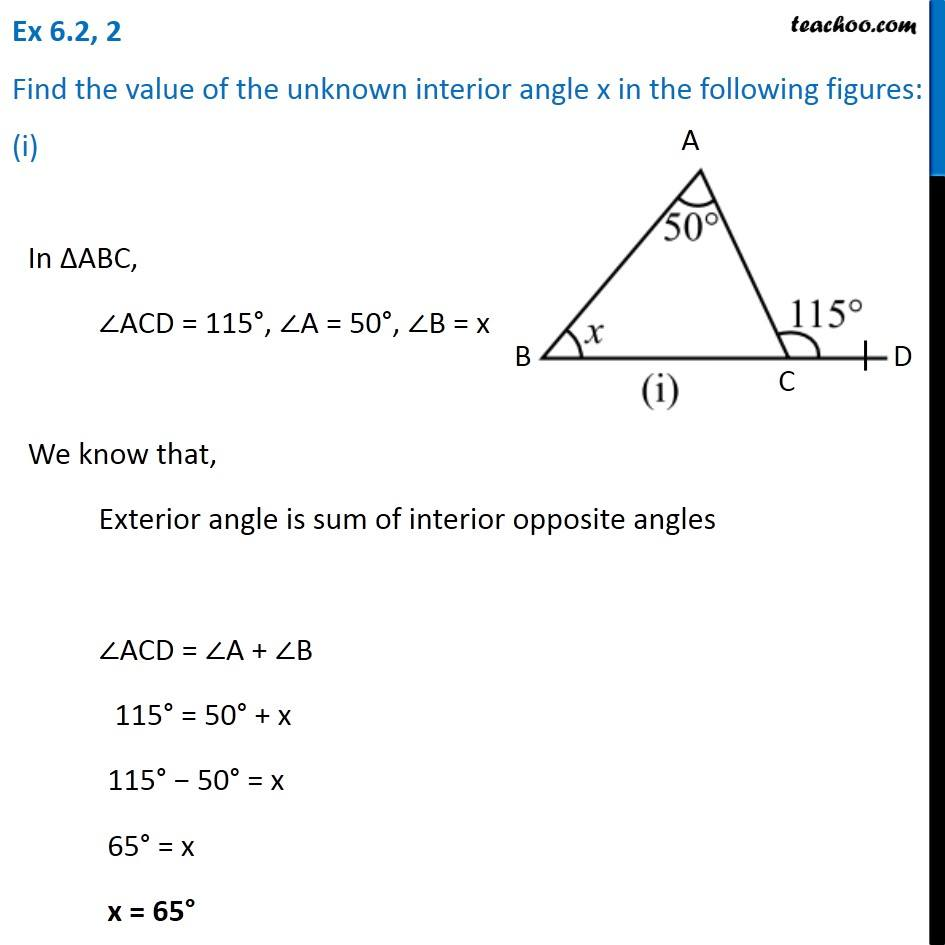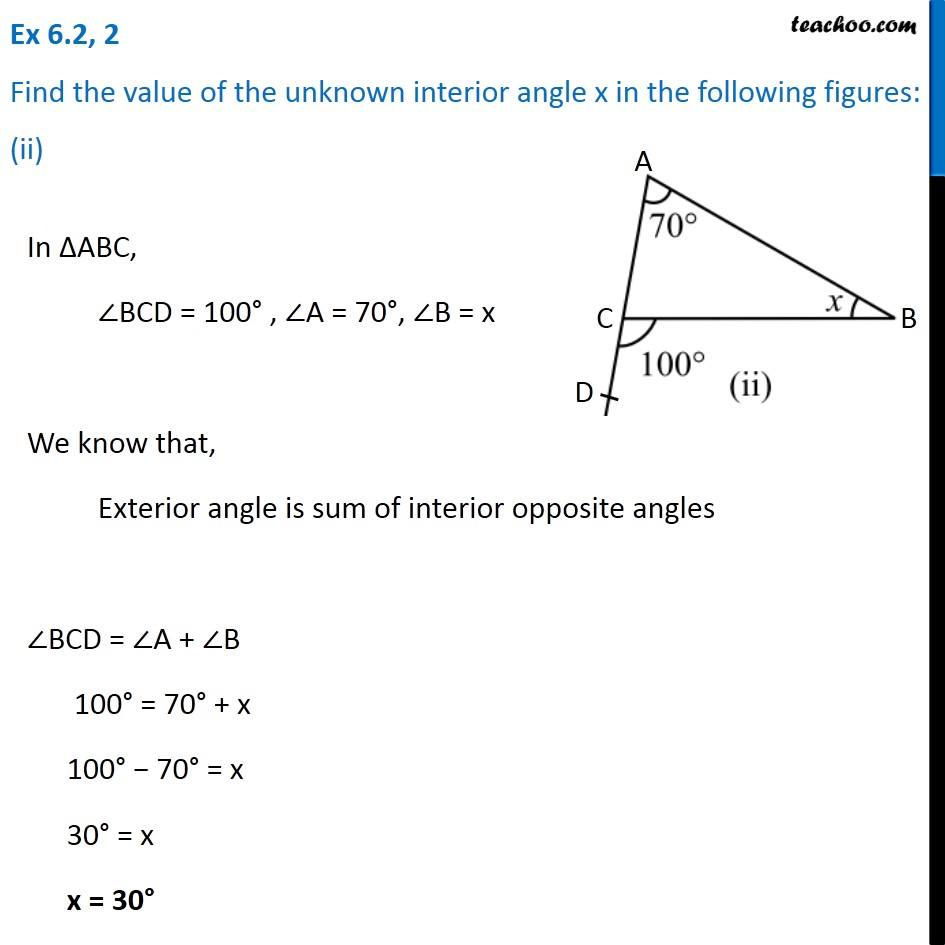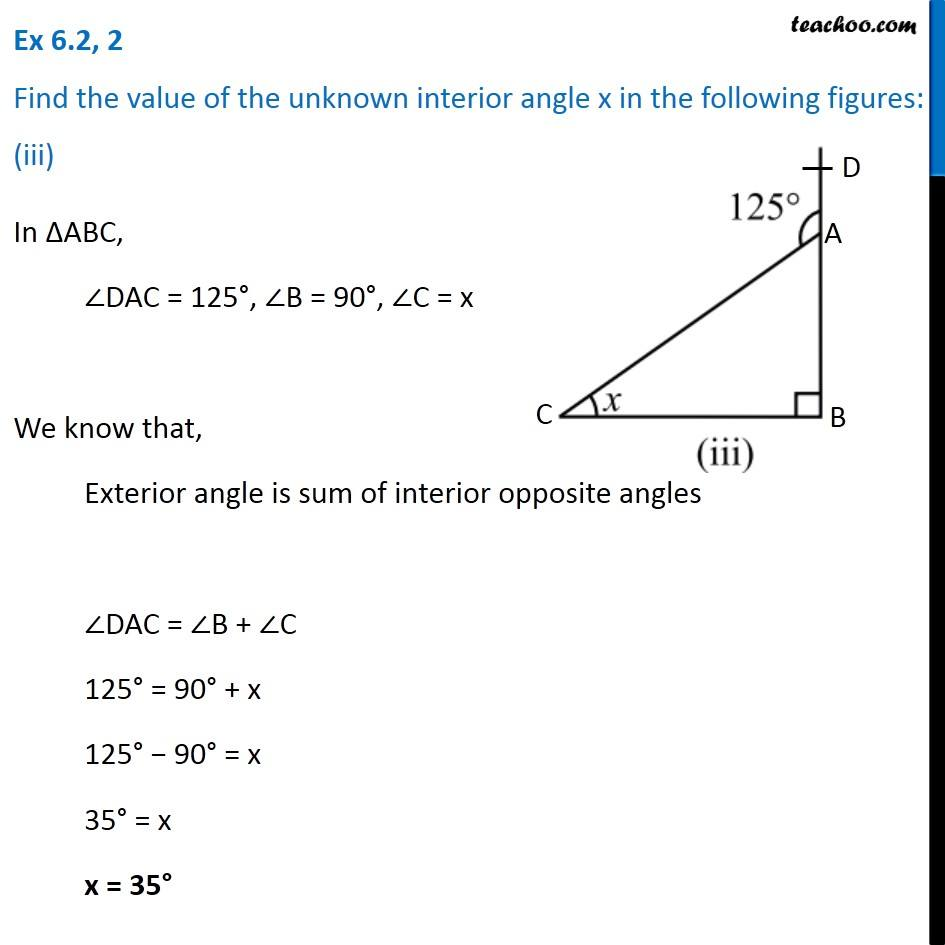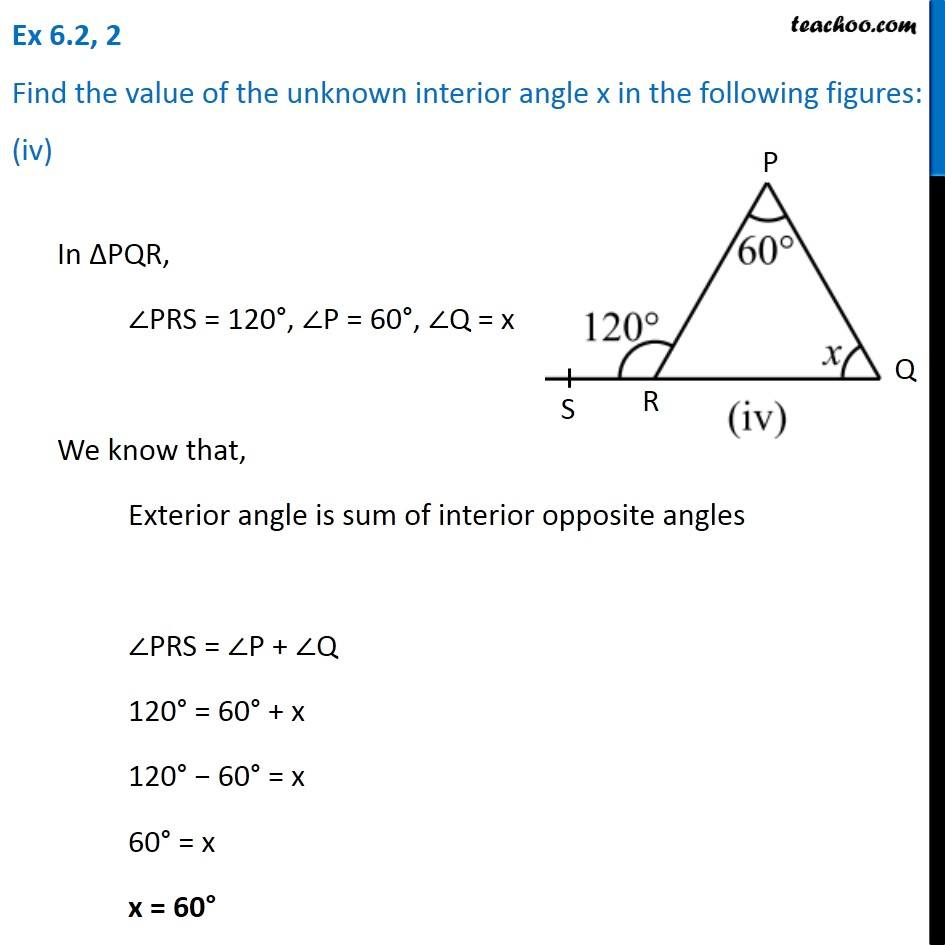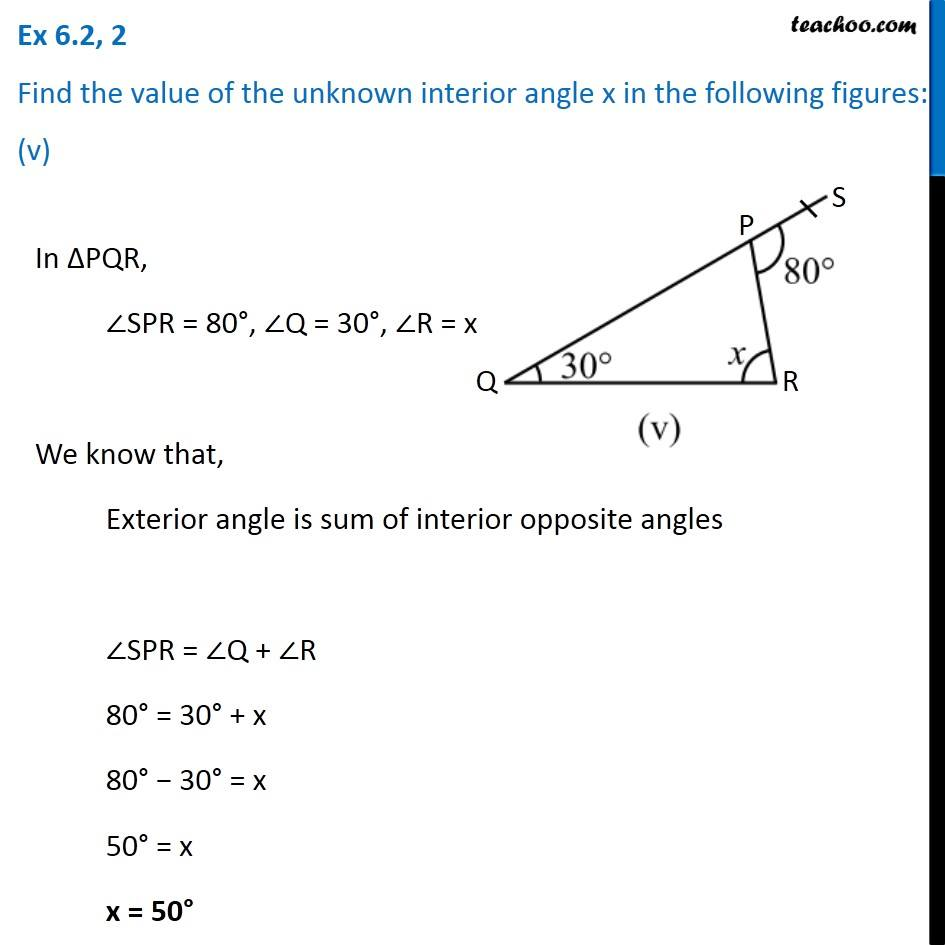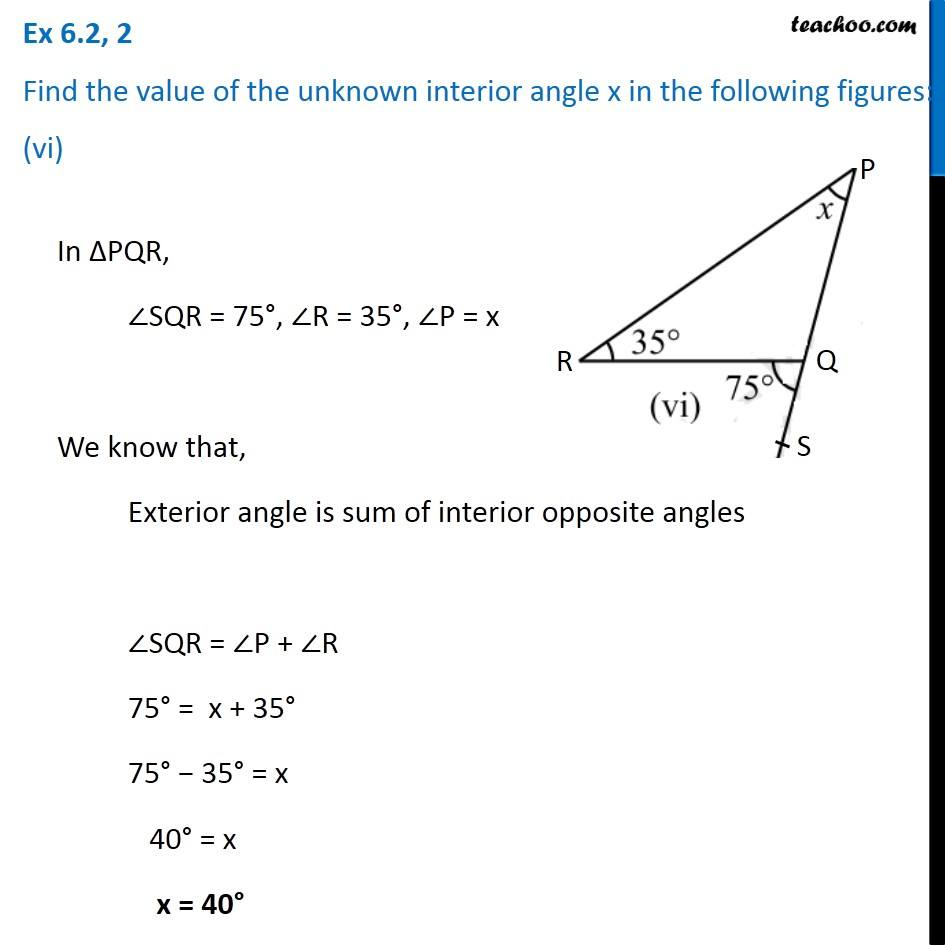1. Chapter 6 Class 7 Triangle and its Properties
2. Concept wise
3. Exterior angles of a triangle

Transcript

Ex 6.2, 2 Find the value of the unknown interior angle x in the following figures: (i) In ∆ABC, ∠ACD = 115°, ∠A = 50°, ∠B = x We know that, Exterior angle is sum of interior opposite angles ∠ACD = ∠A + ∠B 115° = 50° + x 115° − 50° = x 65° = x x = 65° Ex 6.2, 2 Find the value of the unknown interior angle x in the following figures: (ii)In ∆ABC, ∠BCD = 100° , ∠A = 70°, ∠B = x We know that, Exterior angle is sum of interior opposite angles ∠BCD = ∠A + ∠B 100° = 70° + x 100° − 70° = x 30° = x x = 30° Ex 6.2, 2 Find the value of the unknown interior angle x in the following figures: (iii)In ∆ABC, ∠DAC = 125°, ∠B = 90°, ∠C = x We know that, Exterior angle is sum of interior opposite angles ∠DAC = ∠B + ∠C 125° = 90° + x 125° − 90° = x 35° = x x = 35° Ex 6.2, 2 Find the value of the unknown interior angle x in the following figures: (iv)In ∆PQR, ∠PRS = 120°, ∠P = 60°, ∠Q = x We know that, Exterior angle is sum of interior opposite angles ∠PRS = ∠P + ∠Q 120° = 60° + x 120° − 60° = x 60° = x x = 60° Ex 6.2, 2 Find the value of the unknown interior angle x in the following figures: (v)In ∆PQR, ∠SPR = 80°, ∠Q = 30°, ∠R = x We know that, Exterior angle is sum of interior opposite angles ∠SPR = ∠Q + ∠R 80° = 30° + x 80° − 30° = x 50° = x x = 50° Ex 6.2, 2 Find the value of the unknown interior angle x in the following figures: (vi) In ∆PQR, ∠SQR = 75°, ∠R = 35°, ∠P = x We know that, Exterior angle is sum of interior opposite angles ∠SQR = ∠P + ∠R 75° = x + 35° 75° − 35° = x 40° = x x = 40°

Exterior angles of a triangle

About the AuthorDavneet Singh
Davneet Singh is a graduate from Indian Institute of Technology, Kanpur. He has been teaching from the past 9 years. He provides courses for Maths and Science at Teachoo.